How Cheenta works to ensure student success?
Explore the Back-Story

# To find Trace of a given Matrix : ISI MMA 2018 Question 13# Understand the problem

[/et_pb_text][et_pb_text _builder_version="3.27" text_font="Raleway||||||||" background_color="#f4f4f4" box_shadow_style="preset2" custom_margin="10px||10px" custom_padding="10px|20px|10px|20px" _i="1" _address="0.0.0.1"]If A = \begin{bmatrix} 2&i\\i&0\end{bmatrix} , the trace of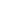is[/et_pb_text][/et_pb_column][/et_pb_row][et_pb_row _builder_version="3.25" _i="1" _address="0.1"][et_pb_column type="4_4" _builder_version="3.25" custom_padding="|||" custom_padding__hover="|||" _i="0" _address="0.1.0"][et_pb_accordion open_toggle_text_color="#0c71c3" _builder_version="3.27" toggle_font="||||||||" body_font="Raleway||||||||" text_orientation="center" custom_margin="10px||10px" _i="0" _address="0.1.0.0"][et_pb_accordion_item title="Source of the problem" open="on" _builder_version="3.27" hover_enabled="0" _i="0" _address="0.1.0.0.0"]Sample Questions (MMA):2019[/et_pb_accordion_item][et_pb_accordion_item title="Topic" _builder_version="3.27" hover_enabled="0" _i="1" _address="0.1.0.0.1" open="off"]Linear Algebra[/et_pb_accordion_item][et_pb_accordion_item title="Difficulty Level" _builder_version="3.27" hover_enabled="0" _i="2" _address="0.1.0.0.2" open="off"]Medium[/et_pb_accordion_item][et_pb_accordion_item title="Suggested Book" _builder_version="3.27" hover_enabled="0" _i="3" _address="0.1.0.0.3" open="off"]Introduction to Linear Algebra by Pearson[/et_pb_accordion_item][/et_pb_accordion][et_pb_text _builder_version="3.23.3" text_font="Raleway|300|||||||" text_text_color="#ffffff" header_font="Raleway|300|||||||" header_text_color="#e2e2e2" background_color="#0c71c3" border_radii="on|5px|5px|5px|5px" box_shadow_style="preset3" custom_margin="48px||48px" custom_padding="20px|20px|20px|20px" _i="1" _address="0.1.0.1"]

[/et_pb_text][et_pb_tabs active_tab_background_color="#0c71c3" inactive_tab_background_color="#000000" _builder_version="3.27" tab_text_color="#ffffff" tab_font="||||||||" background_color="#ffffff" hover_enabled="0" _i="2" _address="0.1.0.2"][et_pb_tab title="Hint 0" _builder_version="3.22.4" _i="0" _address="0.1.0.2.0"]Do you really need a hint? Try it first!

[/et_pb_tab][et_pb_tab title="Hint 1" _builder_version="3.27" hover_enabled="0" _i="1" _address="0.1.0.2.1"]A = \begin{pmatrix} 2&i\\i&0 \end{pmatrix} ;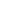= \begin{pmatrix} 3&2i\\2i&-1 \end{pmatrix}= \begin{pmatrix} 4&3i\\i&-2 \end{pmatrix} Now , we can observe that the trace is always going to be 2 . Now we're to seal the deal[/et_pb_tab][et_pb_tab title="Hint 2" _builder_version="3.27" hover_enabled="0" _i="2" _address="0.1.0.2.2"]One way to think about this problem is to go into eigen values as the tr(A) .( Eigenvalues are a special set of scalars associated with a linear system of equations (i.e., a matrix equation) that are sometimes also known as characteristic roots, characteristic values  and  proper values, or latent roots .) (Eigenvectors are a special set of vectors associated with a linear system of equations (i.e., a matrix equation) that are sometimes also known as characteristic vectors, proper vectors, or latent vectors ), remains unaltered in between similar matrices.[/et_pb_tab][et_pb_tab title="Hint 3" _builder_version="3.27" hover_enabled="0" _i="3" _address="0.1.0.2.3"]|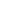I - A | = 0 => \begin{matrix} \lambda -2&-i\\-i&\lambda \end{matrix} =0 =>(- 2) + 1 = 0 =>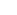- 2+ 1 = 0 =>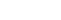= 0 =>= 1,1 So , what will be the canonical form ?[/et_pb_tab][et_pb_tab title="Hint 4" _builder_version="3.27" hover_enabled="0" _i="4" _address="0.1.0.2.4"]So , the canonical  matrix will be \begin{pmatrix} 1&0\\0&1 \end{pmatrix} = A' , i.e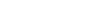o.t A' =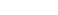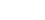=  \begin{pmatrix} 1^{10}&0\\0&1^{10}\end{pmatrix} tr() = tr((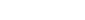) => tr(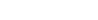=> tr((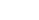)  => (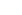) + () => 2(Ans)               [/et_pb_tab][/et_pb_tabs][et_pb_text _builder_version="3.23.3" text_font="Raleway|300|||||||" text_text_color="#ffffff" header_font="Raleway|300|||||||" header_text_color="#e2e2e2" background_color="#0c71c3" border_radii="on|5px|5px|5px|5px" box_shadow_style="preset3" custom_margin="48px||48px" custom_padding="20px|20px|20px|20px" _i="3" _address="0.1.0.3"]

# Understand the problem

[/et_pb_text][et_pb_text _builder_version="3.27" text_font="Raleway||||||||" background_color="#f4f4f4" box_shadow_style="preset2" custom_margin="10px||10px" custom_padding="10px|20px|10px|20px" _i="1" _address="0.0.0.1"]If A = \begin{bmatrix} 2&i\\i&0\end{bmatrix} , the trace ofis[/et_pb_text][/et_pb_column][/et_pb_row][et_pb_row _builder_version="3.25" _i="1" _address="0.1"][et_pb_column type="4_4" _builder_version="3.25" custom_padding="|||" custom_padding__hover="|||" _i="0" _address="0.1.0"][et_pb_accordion open_toggle_text_color="#0c71c3" _builder_version="3.27" toggle_font="||||||||" body_font="Raleway||||||||" text_orientation="center" custom_margin="10px||10px" _i="0" _address="0.1.0.0"][et_pb_accordion_item title="Source of the problem" open="on" _builder_version="3.27" hover_enabled="0" _i="0" _address="0.1.0.0.0"]Sample Questions (MMA):2019[/et_pb_accordion_item][et_pb_accordion_item title="Topic" _builder_version="3.27" hover_enabled="0" _i="1" _address="0.1.0.0.1" open="off"]Linear Algebra[/et_pb_accordion_item][et_pb_accordion_item title="Difficulty Level" _builder_version="3.27" hover_enabled="0" _i="2" _address="0.1.0.0.2" open="off"]Medium[/et_pb_accordion_item][et_pb_accordion_item title="Suggested Book" _builder_version="3.27" hover_enabled="0" _i="3" _address="0.1.0.0.3" open="off"]Introduction to Linear Algebra by Pearson[/et_pb_accordion_item][/et_pb_accordion][et_pb_text _builder_version="3.23.3" text_font="Raleway|300|||||||" text_text_color="#ffffff" header_font="Raleway|300|||||||" header_text_color="#e2e2e2" background_color="#0c71c3" border_radii="on|5px|5px|5px|5px" box_shadow_style="preset3" custom_margin="48px||48px" custom_padding="20px|20px|20px|20px" _i="1" _address="0.1.0.1"]

[/et_pb_text][et_pb_tabs active_tab_background_color="#0c71c3" inactive_tab_background_color="#000000" _builder_version="3.27" tab_text_color="#ffffff" tab_font="||||||||" background_color="#ffffff" hover_enabled="0" _i="2" _address="0.1.0.2"][et_pb_tab title="Hint 0" _builder_version="3.22.4" _i="0" _address="0.1.0.2.0"]Do you really need a hint? Try it first!

[/et_pb_tab][et_pb_tab title="Hint 1" _builder_version="3.27" hover_enabled="0" _i="1" _address="0.1.0.2.1"]A = \begin{pmatrix} 2&i\\i&0 \end{pmatrix} ;= \begin{pmatrix} 3&2i\\2i&-1 \end{pmatrix}= \begin{pmatrix} 4&3i\\i&-2 \end{pmatrix} Now , we can observe that the trace is always going to be 2 . Now we're to seal the deal[/et_pb_tab][et_pb_tab title="Hint 2" _builder_version="3.27" hover_enabled="0" _i="2" _address="0.1.0.2.2"]One way to think about this problem is to go into eigen values as the tr(A) .( Eigenvalues are a special set of scalars associated with a linear system of equations (i.e., a matrix equation) that are sometimes also known as characteristic roots, characteristic values  and  proper values, or latent roots .) (Eigenvectors are a special set of vectors associated with a linear system of equations (i.e., a matrix equation) that are sometimes also known as characteristic vectors, proper vectors, or latent vectors ), remains unaltered in between similar matrices.[/et_pb_tab][et_pb_tab title="Hint 3" _builder_version="3.27" hover_enabled="0" _i="3" _address="0.1.0.2.3"]|I - A | = 0 => \begin{matrix} \lambda -2&-i\\-i&\lambda \end{matrix} =0 =>(- 2) + 1 = 0 =>- 2+ 1 = 0 =>= 0 =>= 1,1 So , what will be the canonical form ?[/et_pb_tab][et_pb_tab title="Hint 4" _builder_version="3.27" hover_enabled="0" _i="4" _address="0.1.0.2.4"]So , the canonical  matrix will be \begin{pmatrix} 1&0\\0&1 \end{pmatrix} = A' , i.eo.t A' ==  \begin{pmatrix} 1^{10}&0\\0&1^{10}\end{pmatrix} tr() = tr(() => tr(=> tr(()  => () + () => 2(Ans)               [/et_pb_tab][/et_pb_tabs][et_pb_text _builder_version="3.23.3" text_font="Raleway|300|||||||" text_text_color="#ffffff" header_font="Raleway|300|||||||" header_text_color="#e2e2e2" background_color="#0c71c3" border_radii="on|5px|5px|5px|5px" box_shadow_style="preset3" custom_margin="48px||48px" custom_padding="20px|20px|20px|20px" _i="3" _address="0.1.0.3"]

# Similar Problems

This site uses Akismet to reduce spam. Learn how your comment data is processed.

### Knowledge Partner